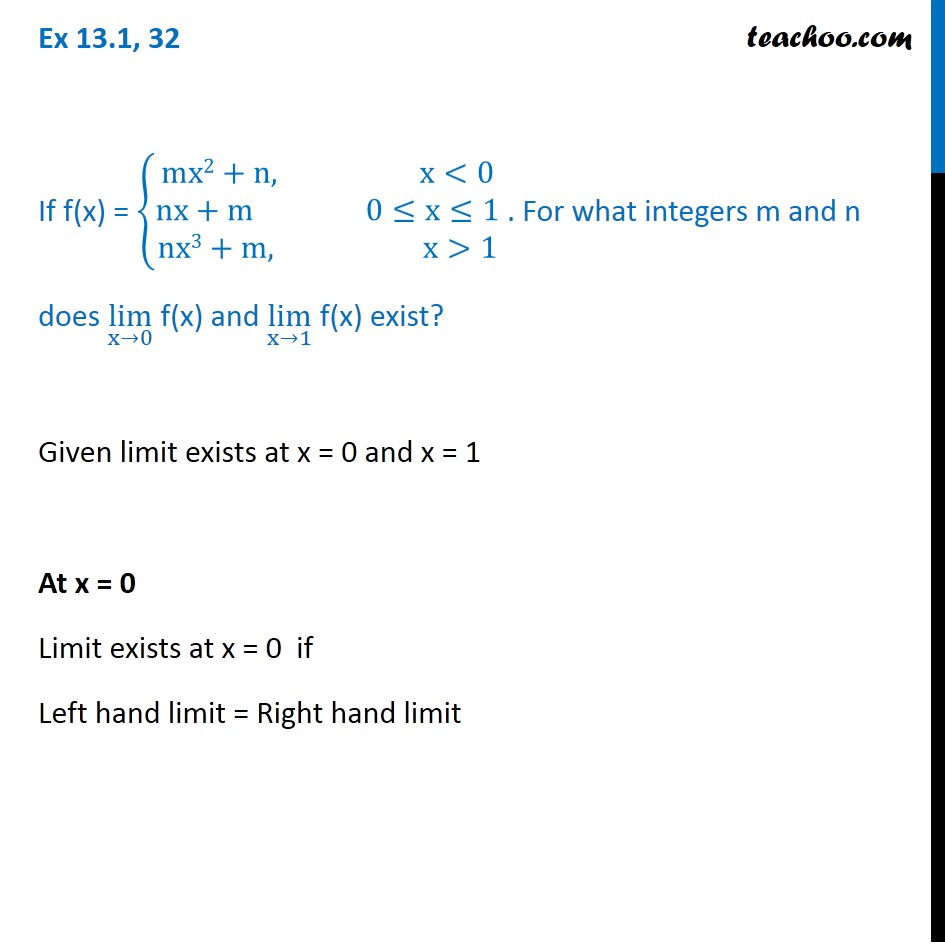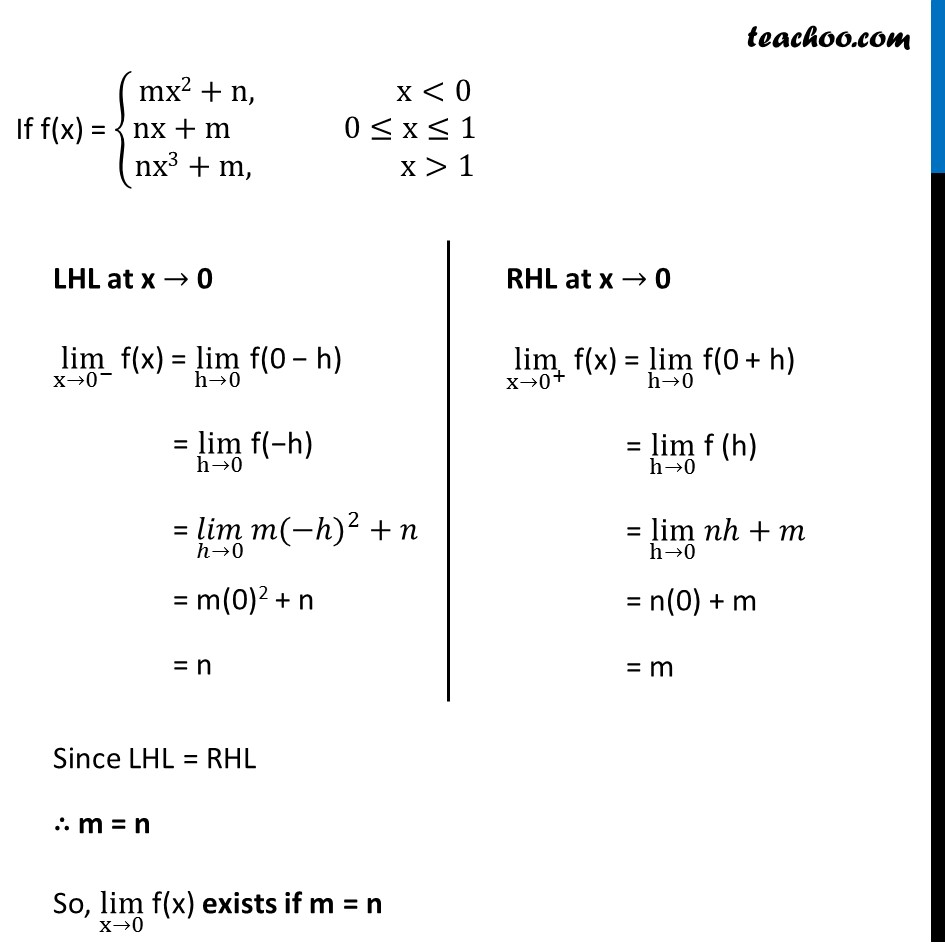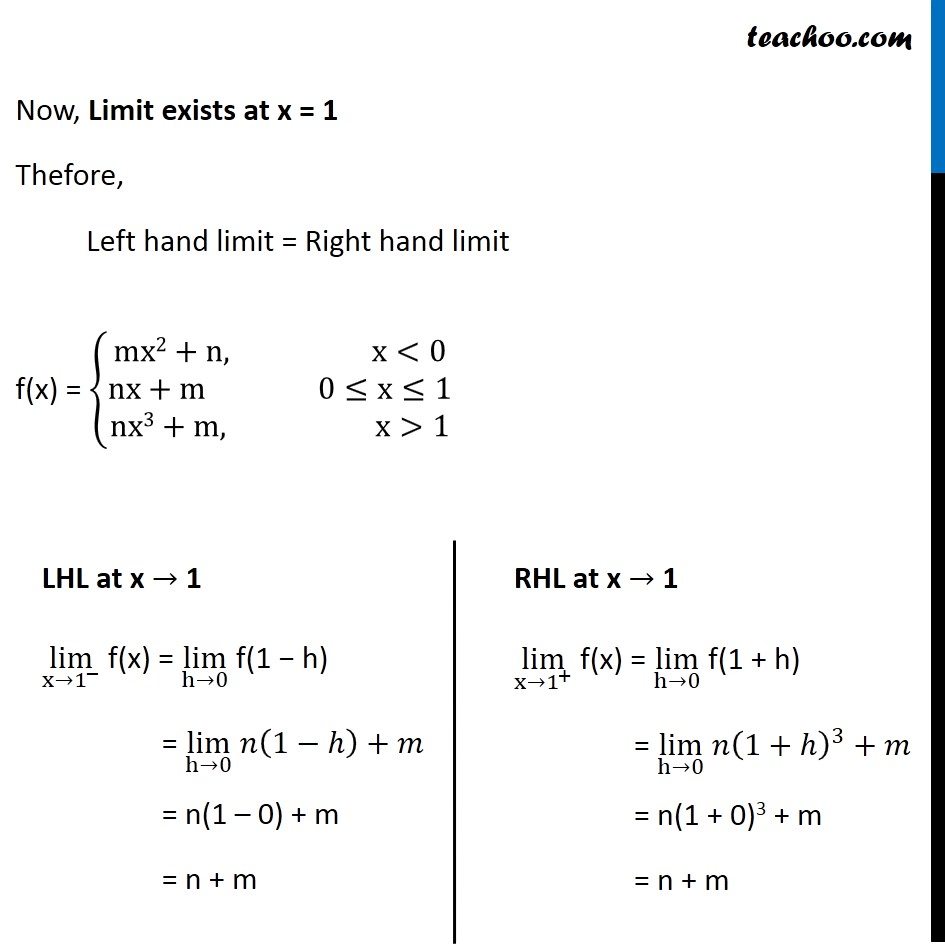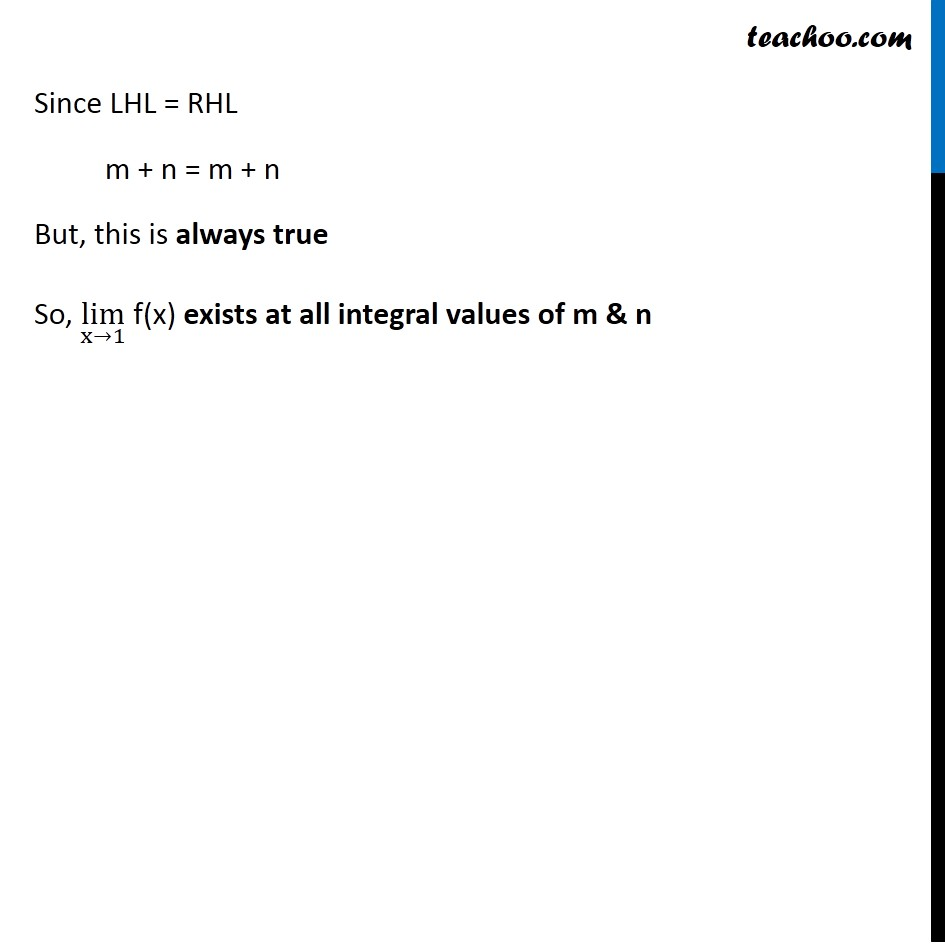1. Chapter 13 Class 11 Limits and Derivatives (Term 1 and Term 2)
2. Concept wise
3. Limits - Limit exists

Transcript

Ex 13.1, 32 If f(x) = {█(mx2+n, x<0@nx+m 0≤x≤1@nx3+m, x>1)┤ . For what integers m and n does lim┬(x→0) f(x) and lim┬(x→1) f(x) exist? Given limit exists at x = 0 and x = 1 At x = 0 Limit exists at x = 0 if Left hand limit = Right hand limit If f(x) = {█(mx2+n, x<0@nx+m 0≤x≤1@nx3+m, x>1)┤ LHL at x → 0 lim┬(x→0^− ) f(x) = lim┬(h→0) f(0 − h) = lim┬(h→0) f(−h) = lim┬(h→0) mh^2+n = m(0)2 + n = n RHL at x → 0 lim┬(x→0^+ ) f(x) = lim┬(h→0) f(0 + h) = lim┬(h→0) f (h) = lim┬(h→0) 𝑛ℎ+𝑚 = n(0) + m = m Since LHL = RHL ∴ m = n So, lim┬(x→0) f(x) exists if m = n Now, Limit exists at x = 1 Thefore, Left hand limit = Right hand limit f(x) = {█(mx2+n, x<0@nx+m 0≤x≤1@nx3+m, x>1)┤ LHL at x → 1 lim┬(x→1^− ) f(x) = lim┬(h→0) f(1 − h) = lim┬(h→0) 𝑛(1−ℎ)+𝑚 = n(1 – 0) + m = n + m RHL at x → 1 lim┬(x→1^+ ) f(x) = lim┬(h→0) f(1 + h) = lim┬(h→0) 𝑛(1+ℎ)^3+𝑚 = n(1 + 0)3 + m = n + m Since LHL = RHL m + n = m + n But, this is always true So, lim┬(x→1) f(x) exists at all integral values of m & n